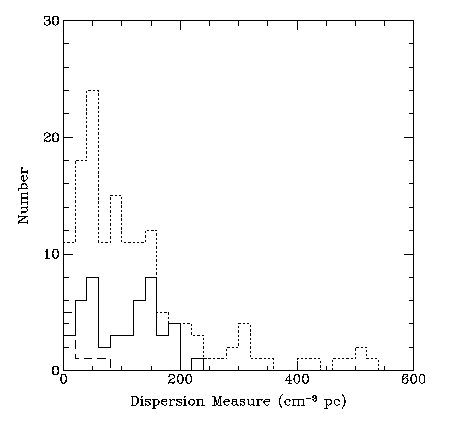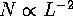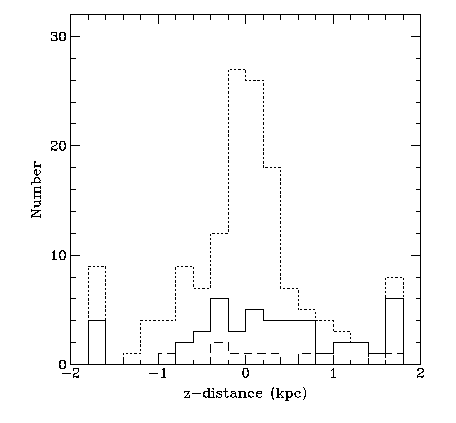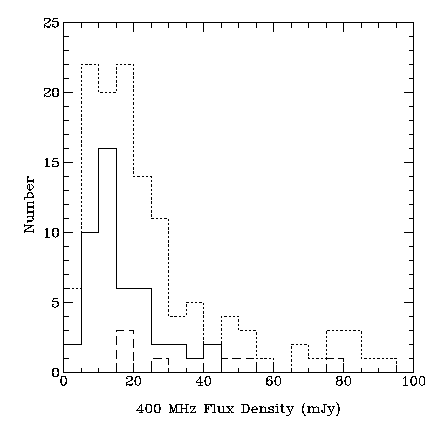Next: The derived luminosity and Up: The galactic population of Previous: The galactic population of

## The observed distributionFigure 16: The observed distribution of pulsar dispersion measures. Dotted curve is the total known population, solid curve is the Parkes survey.Figure 17: The observed distribution of pulsar distances above the galactic plane. Dotted curve is the total known population, solid curve is the Parkes survey.Figure 18: The observed distribution of pulsar flux densities. Dotted curve is the total known population, solid curve is the Parkes survey.

Jon Bell
Thu Dec 19 15:15:11 GMT 1996